# Work, Energy and Power

Work, Energy and Power are fundamental concepts of Physics. Work is said to be done when a force (push or pull) applied to an object causes a displacement of the object. We define the capacity to do the work as energy. Power is the work done per unit of time. This article discusses work, energy and power in detail.

## What is Work?

For work to be done, a force must be exerted and there must be motion or displacement in the direction of the force. The work done by a force acting on an object is equal to the magnitude of the force multiplied by the distance moved in the direction of the force. Work has only magnitude and no direction. Hence, work is a scalar quantity.

### Formula of Work

The work done by a force is defined to be the product of the component of the force in the direction of the displacement and the magnitude of this displacement.

$$\begin{array}{l}W=F\cos\Theta=\vec{F}\vec{d}\end{array}$$

Where W is the work done, F is the force, d is the displacement, θ is the angle between force and displacement and F cosθ is the component of force in the direction of displacement.

We understand from the work equation that if there is no displacement, there is no work done, irrespective of how large the force is. To summarize, we can say that no work is done if:

• the displacement is zero
• the force is zero
• the force and displacement are mutually perpendicular to each other.

#### Can the work done be negative? Watch the video and find out!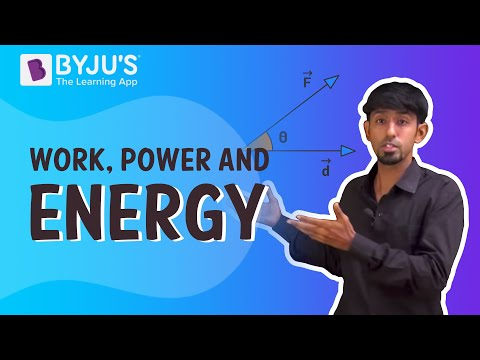### Unit of Work

The SI unit of work is Joule (J). For example, if a force of 5 newtons is applied to an object and moves 2 meters, the work done will be 10 newton-meter or 10 Joule. It should be noted that 1 J = 1 N ⋅ m = 1 kg ⋅ m2/s2.

### Example of Work

An object is horizontally dragged across the surface by a 100 N force acting parallel to the surface. Find out the amount of work done by the force in moving the object through a distance of 8 m.

Solution:

Given:

F = 100 N, d = 8 m

Since F and d are in the same direction, θ = 0, [θ is the angle of the force to the direction of movement], therefore

W = FdCos θ

W = 100 x 8 x Cos 0

W = 800 J [Since Cos 0 = 1]

## What is Energy?

Energy is the ability to perform work. Energy can neither be created nor destroyed, and it can only be transformed from one form to another. The unit of Energy is the same as of Work, i.e. Joules. Energy is found in many things, and thus there are different types of energy.

All forms of energy are either kinetic or potential. The energy in motion is known as Kinetic Energy, whereas Potential Energy is the energy stored in an object and is measured by the amount of work done.

### Types of Energy

Some other types of energy are given below:

• Mechanical energy
• Mechanical wave energy
• Chemical energy
• Electric energy
• Magnetic energy
• Nuclear energy
• Ionization energy
• Elastic energy
• Gravitational energy
• Thermal energy
• Heat Energy

### Unit of Energy

The SI unit of energy is Joules (J), named in honour of James Prescott Joule.

## Watch the video and solve NCERT exercise questions in the chapter Work and Energy Class 9## What is Power?

Power is a physical concept with several different meanings, depending on the context and the available information. We can define power as the rate of doing work, and it is the amount of energy consumed per unit of time.

### Formula of Power

As discussed, power is the rate of doing work. Therefore, it can be calculated by dividing work done by time. The formula for power is given below.

 $$\begin{array}{l}P = \frac{W}{t}\end{array}$$

Where, P is the power, W is the work done and t is the time taken.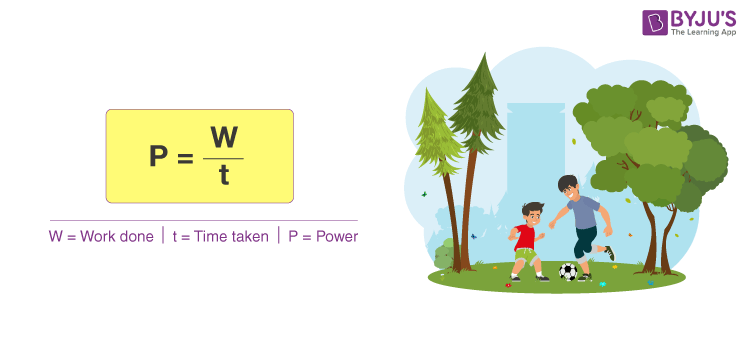### Unit of Power

As power doesn’t have any direction, it is a scalar quantity. The SI unit of power is Joules per Second (J/s), which is termed as Watt. Watt can be defined as the power needed to do one joule of work in one second. The unit Watt is dedicated in honour of Sir James Watt, the developer of the steam engine.

Read the article below to learn the SI unit of power in detail.

### Example of Power

A garage hoist lifts a truck up 2 meters above the ground in 15 seconds. Find the power delivered to the truck. [Given: 1000 kg as the mass of the truck]

First we need to calculate the work done, which requires the force necessary to lift the truck against gravity:

F = mg = 1000 x 9.81 = 9810 N.

W = Fd = 9810N x 2m = 19620 Nm = 19620 J.

The power is P = W/t = 19620J / 15s = 1308 J/s = 1308 W.

### Work, Power and Energy Questions

1. What is the relationship between work, energy and power?
2. What happens to the energy as work is done?
3. What is the difference between work, energy and power?
4. Is energy transferred the same as work done?
5. How does work affect an object’s energy?
6. How are work, energy and power related to each other?
7. How are force, energy and work related?
8. What is the formula of work, energy and power?
9. How do you calculate energy from power?
10. Can force be converted into energy?

## Video Explanation of Work, Energy and Power

Here is an engaging video explanation of Work, Energy and Power and the relationship between them.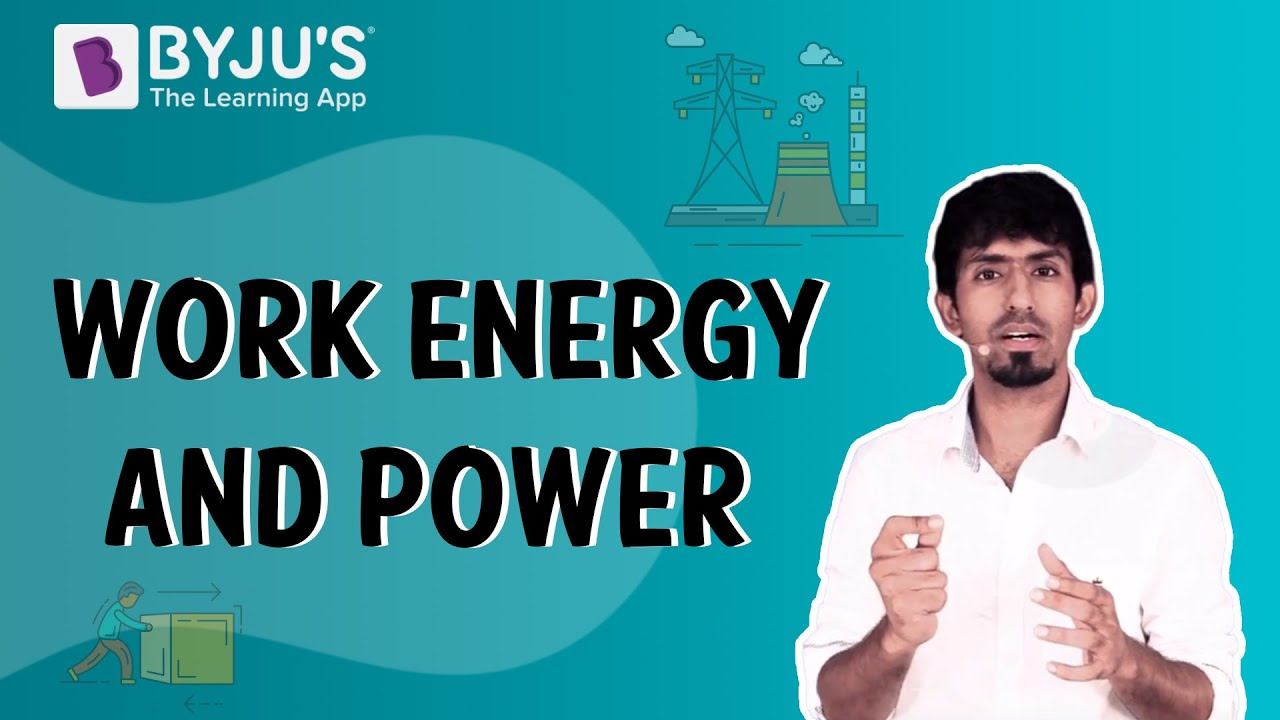## Overview of Work, Energy and Power

### Work

Definition The work done by a force is defined to be the product of component of the force in the direction of the displacement and the magnitude of this displacement.
Formula Work can be calculated by multiplying Force and Distance in the direction of force as follows

W = F × d

Unit The SI unit of work is the Joule (J)

### Energy

Definition Energy is defined as the capacity to do work.
Formula The energy stored in an object due to its position and height is known as potential energy and is given by the formula:

P.E. = mgh

Unit The SI unit of energy is Joules (J).

### Power

Definition Power is defined as the rate at which work is done.
Formula The formula for power is

P = W/t

Unit The SI unit of power is Watt (W).

## Watch the video and solve complete exemplar questions in the chapter Work and Energy Class 9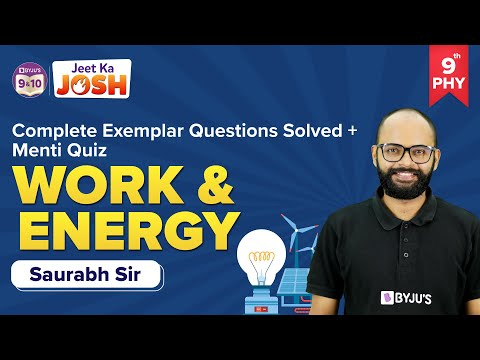Q1

### How are work, energy and power related to each other?

Work is the energy needed to apply a force to move an object a particular distance. Power is the rate at which that work is done.

Q2

### What is the unit of work?

The unit of work is Joule.

Q3

### What is the unit of energy?

The unit of energy is Joule.

Q4

### What is the unit of power?

The unit of power is Watt.

Q5

### Is power a scalar quantity?

Power is a scalar quantity because it is a ratio of two scalar quantities.

Watch this fun and engaging rapid fire session based on the topic Work, Energy and Power!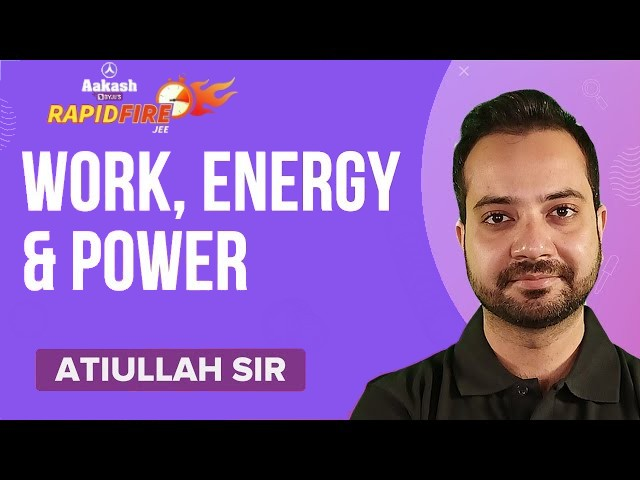Work, Power and Energy is one of the important topics of JEE Main and JEE Advanced, watch the video and understand the type of questions asked from this topic!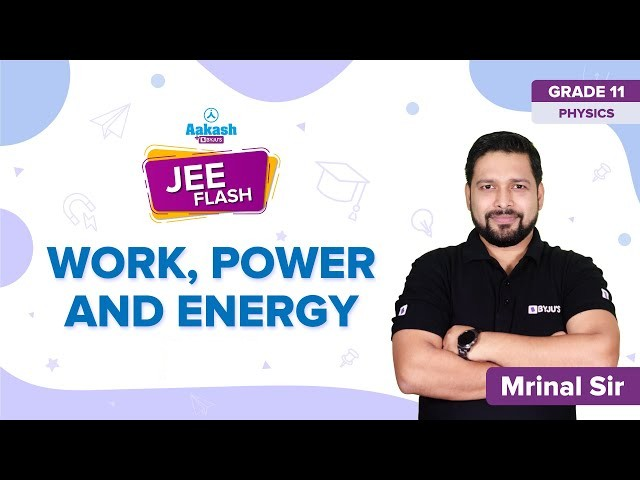Stay tuned to BYJU’S and Fall in Love with Learning!

Test your Knowledge on Work Energy Power

1. Lovely

• Its so useful love this byju’s

• 👍

2. Very good explanation

3. thanks

4. nice

5. Good

Good

6. Excellent explaination done
5 stars to BYJU’S

7. it is very nice because it become easy.

8. Enjoyable

9. Very nice

10. very nicely done

11. well explained

12. really good platform to learn basic

13. Thanks a lot . It was very helpful . Lots of love for all your team .

14. I really love this explanation

15. Thank you, this explanation is very useful for this topic.

16. Amazing! Today was my online class test, the content given here helped me a lot. I would like to give BYJU’S five stars.

17. Good explanation 👍👍👍👍👍👍

18. Really good explanation
It was very useful
Thank you so much

19. What a nice explanation!